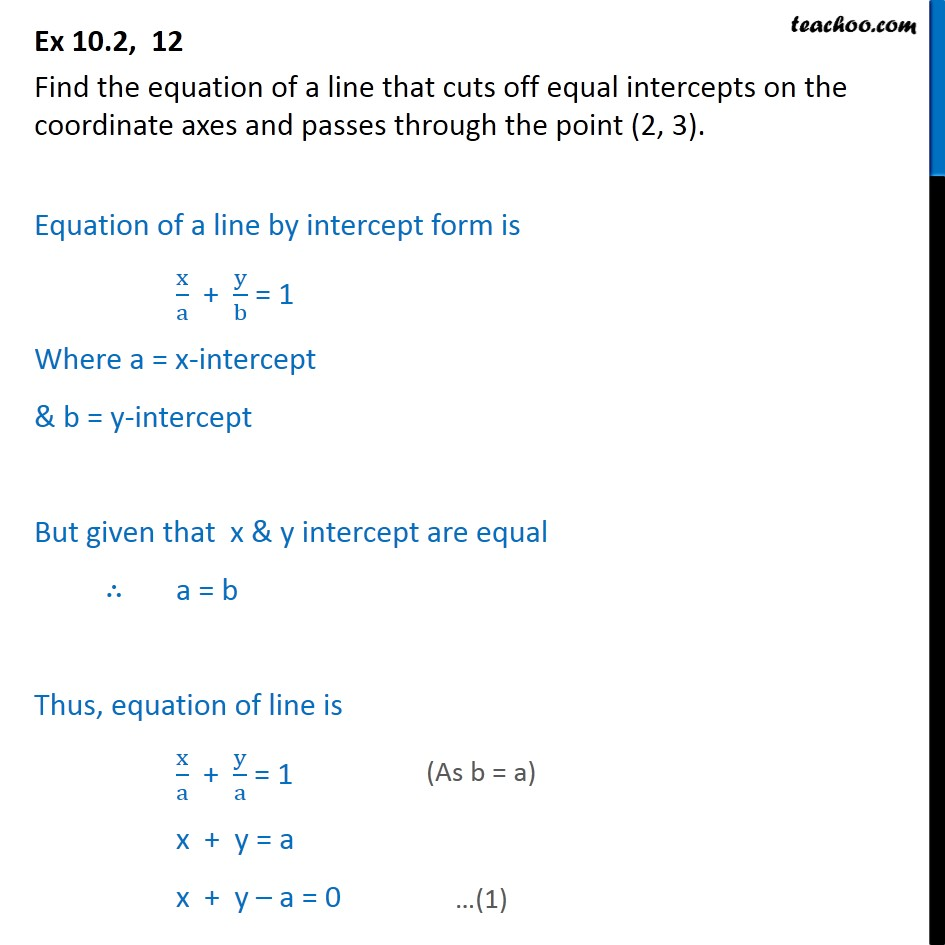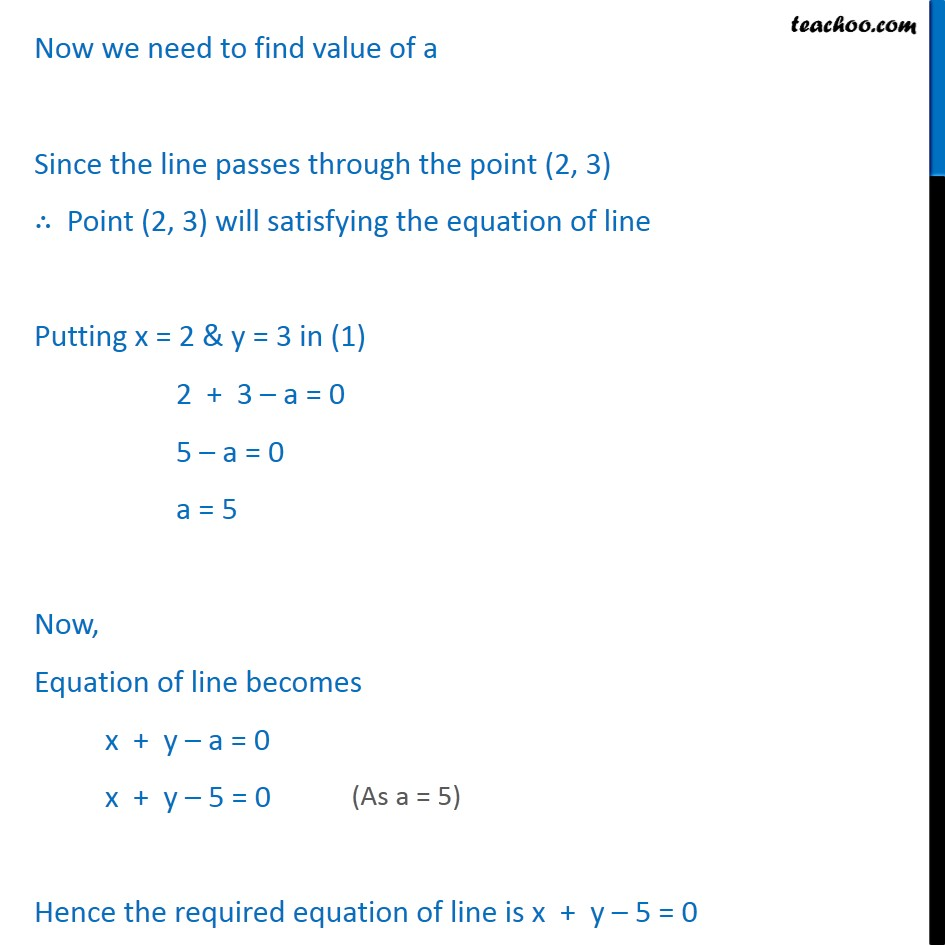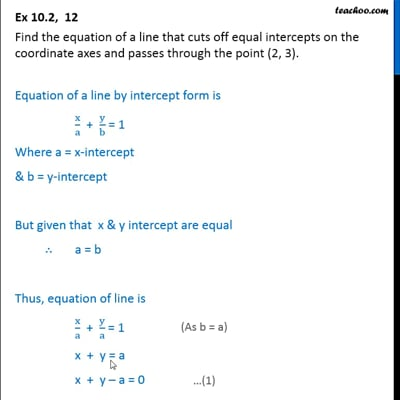Ex 10.2

Chapter 10 Class 11 Straight Lines (Term 1)
Serial order wiseThis video is only available for Teachoo black users

### Transcript

Ex 10.2, 12 Find the equation of a line that cuts off equal intercepts on the coordinate axes and passes through the point (2, 3). Equation of a line by intercept form is x/a + y/b = 1 Where a = x-intercept & b = y-intercept But given that x & y intercept are equal a = b Thus, equation of line is x/a + y/a = 1 x + y = a x + y a = 0 Now we need to find value of a Since the line passes through the point (2, 3) Point (2, 3) will satisfying the equation of line Putting x = 2 & y = 3 in (1) 2 + 3 a = 0 5 a = 0 a = 5 Now, Equation of line becomes x + y a = 0 x + y 5 = 0 Hence the required equation of line is x + y 5 = 0